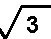## Friday, April 9, 2010

### Assignment-Polynomials

Q1.Find the zeroes of the following quadratic polynomial and verify the relationship between the zeroes and the coefficients:
(1) 4x^2– 4x – 3
(2) 2x^2 – 1
(3) 6x^2 -3-7x

Q2. Find the quadratic polynomial, the sum and product of whose zeroes are
(1) ½ , -2
(2) –3, -7

Q3. Find all the zeros of x^4 + 3x^3 – 20x^2 – 6x + 36, if two of it’s zeroes areand -Q4. Write the zeroes of the polynomial x^2 – x – 6.
Q5. Write a quadratic polynomial whose zeroes are 5 and -5.
Q6. The graph of y=f(x) is given below .Find the number of zeroes and the zeroes of f(x):Q7. The graph of y=f(x) is shown below. Find the number of zeros of f(x).Q8.If the polynomial 6x^4 +8x^3 +17x^2+21x+7 is divided by another polynomial 3x^2+4x+1, the remainder comes out to be ax +b , find a and b.
Q9. Write the general form of quadratic polynomial.
Q10. Write the quadratic polynomial whose zeroes are 2and 2.
Q11.Give an example of polynomial f(x),g(x),q(x) and r(x) satisfying
f(x)=g(x) q(x)+r(x) where degree r(x)=0.
Q12.If the fourth degree polynomial is divided by a quadratic polynomial, write the degree of the remainder.
Q13. Find all the zeros of x^4 + x^3 – 34x^2 – 4x + 120, if two of it’s zeroes are 2 and - 2.